U.S. Department of Transportation
1200 New Jersey Avenue, SE
Washington, DC 20590
202-366-4000

Federal Highway Administration Research and Technology
Coordinating, Developing, and Delivering Highway Transportation InnovationsThis report is an archived publication and may contain dated technical, contact, and link information
 Federal Highway Administration > Publications > Research Publications > 04124 > Lab & Field Testing of AUT Systems for Steel Highway Bridges
Publication Number: FHWA-HRT-04-124
Date: April 2005

# Lab & Field Testing of AUT Systems for Steel Highway Bridges

### 6. RESULTS (cont'd)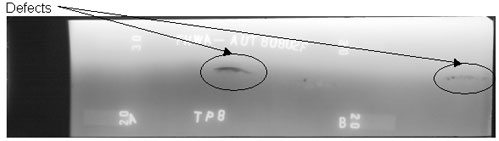Figure 58. Laboratory specimen S135: Radiographic image showing discontinuities in the weld between markers A and B.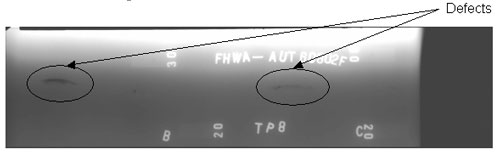Figure 59. Laboratory specimen S135: Radiographic image showing discontinuities in the weld between markers B and C.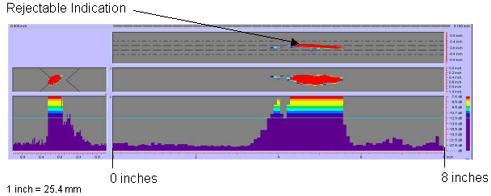Figure 60. P-scan images of laboratory specimen S135: From TSC side of centerline between 0 and 203.2 mm (0 and 8 inches).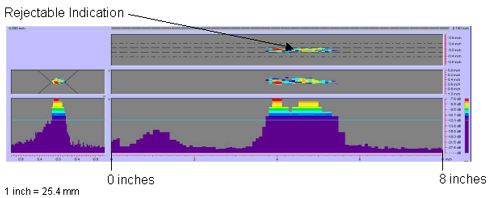Figure 61. P-scan images of laboratory specimen S135: From BSC side of centerline between 0 and 203.2 mm (0 and 8 inches).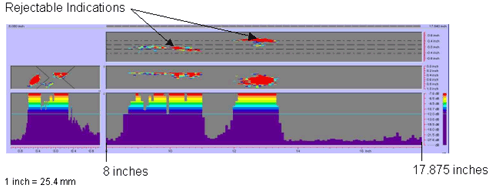Figure 62. P-scan images of laboratory specimen S135: From TSC side of centerline between 203.2 and 454 mm (8 and 17.875 inches).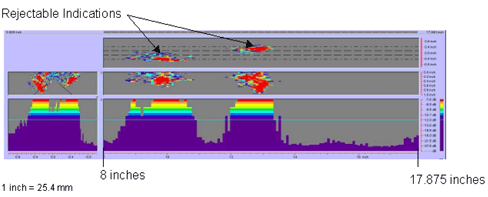Figure 63. P-scan images of laboratory specimen S135: From BSC side of centerline between 203.2 and 454 mm (8 and 17.875 inches).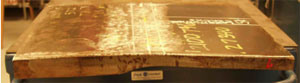Figure 64. Laboratory specimen S136: Side view of joint.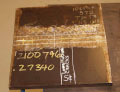Figure 65. Laboratory specimen S136: Top view of joint.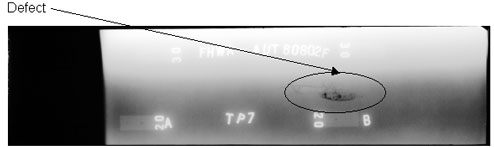Figure 66. Laboratory specimen S136: Radiographic image showing discontinuities in the weld between markers A and B.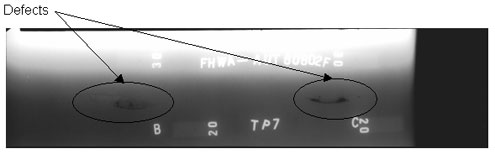Figure 67. Laboratory specimen S136: Radiographic image showing discontinuities in the weld between markers B and C.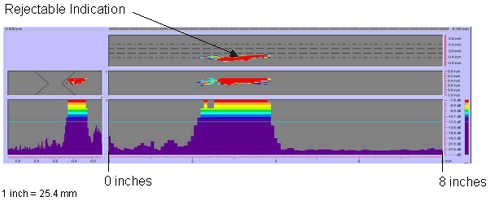Figure 68. P-scan images of laboratory specimen S136: From TSC side of centerline between 0 and 203.2 mm (0 and 8 inches).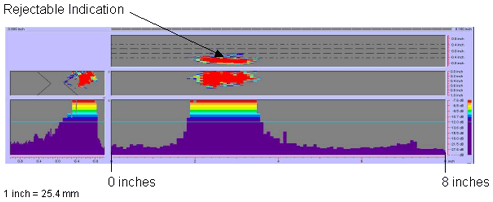Figure 69. P-scan images of laboratory specimen S136: From BSC side of centerline between 0 and 203.2 mm (0 and 8 inches).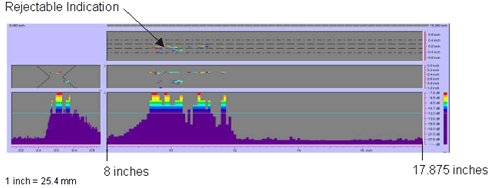Figure 70. P-scan images of laboratory specimen S136: From TSC side of centerline between 203.2 and 454 mm (8 and 17.875 inches).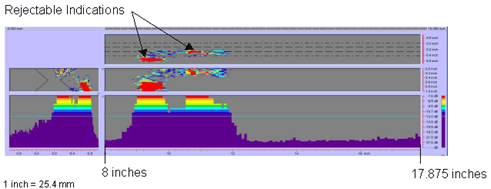Figure 71. P-scan images of laboratory specimen S136: From BSC side of centerline between 203.2 and 454 mm (8 and 17.875 inches).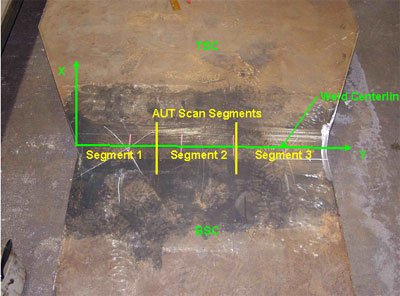Figure 72. Field specimen FG38k-TF2-TopF-FCM used in blind testing: Top view of joint.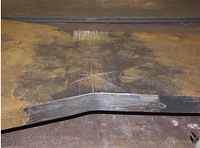Figure 73. Field specimen FG38K-TF2-TopF-FCM used in blind testing: Side view of joint.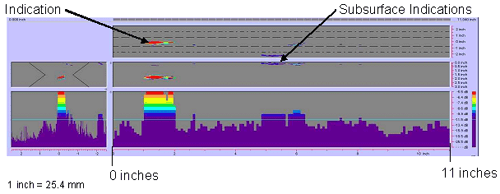Figure 74. P-scan images of field specimen FG38K-TF2-TopF-FCM using 45-degree probe: From TSC side of certerline between 0 and 279.4 mm (0 and 11 inches).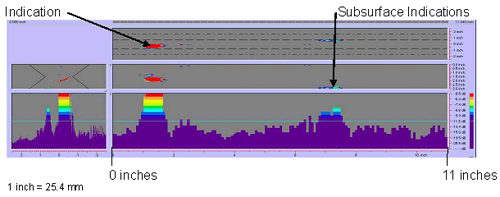Figure 75. P-scan images of field specimen FG38K-TF2TopF-FCM using 45-degree probe: From BSC side of centerline between 0 and 270.4mm (0 and 11 inches).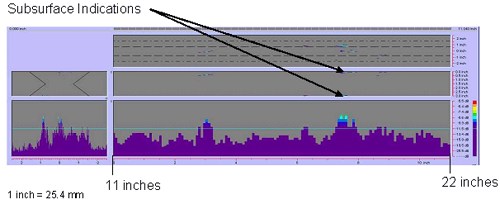Figure 76. P-scan image of field specimen FG38K-TF2-TopF-FCM using 45-degree probe: From TSC side of centerline between 279.4 and 558.8 mm (11 and 22 inches).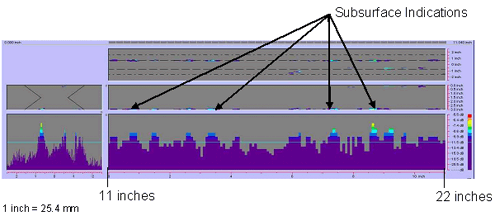Figure 77. P-scan iamges of field specimen FG38K-TF2-TopF-FCM using 45-degree probe: From BSC side of centerline between 279.4 and 558.8 mm (11 and 22 inches).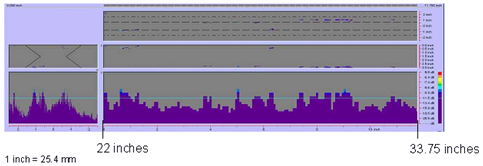Figure 78. P-scan iamges of field specimen FG38K-TF2-TopF-FCM using 45-degree probe: From TSC side of centerline between 558.8 and 857.25 mm (22 and 33.75 inches).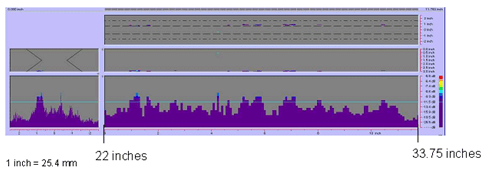Figure 79. P-scan iamges of field specimen FG38K-TF2-TopF-FCM using 45-degree probe: From BSC side of centerline between 558.8 and 857.25 mm (22 and 33.75 inches).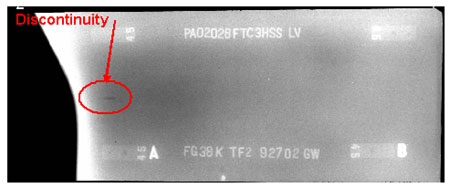Figure 80. Radiographic image of field specimen FG38K-TF2-TopF-TCM: Section A-B.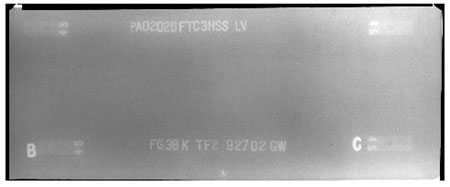Figure 81. Radiographic image of field specimen FG38K-TF2-TopF-FCM: Section B-C.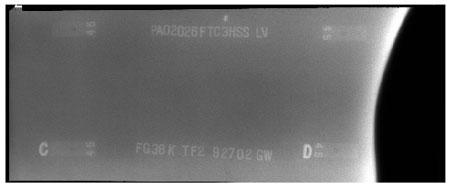Figure 82. Radiographic image of field specimen FG38K-TF2-TopF-FCM: Section C-D.

### BLIND FIELD-TESTING RESULTS

During the course of the AUT field study, it was not convenient to conduct blind testing because of fabrication plant logistics and scheduling. However, one blind test was conducted successfully. The purpose of blind testing was to eliminate bias by performing AUT inspection before RT and manual UT inspection.

The specimen (selected at random) for blind testing was FG38K-TopF-FCM. Specimen FG38K-TopF-FCM was composed of two welded butt joints designated as FG38K-TF1-TopF-FCM and FG38K-TF2-TopF-FCM, each having a width transition. Specimen FG38K-TopF-FCM was 76.2 mm (3 inches) thick, 857.25 mm (33.75 inches) wide, and was designated as a fracture-critical member (FCM). Plates designed as FCMs must be inspected by both RT and manual UT according to section 6.7.1.2 of the AASHTO/AWS D1.5M/D1.5: 2002 Bridge Welding Code.(1) Table 6.2 in the AASHTO/AWS D1.5M/D1.5: 2002 Bridge Welding Code(1) indicates that plates with thicknesses ranging from greater than 60 to 90 mm (2.5 to 3.5 inches) must be inspected by UT using 45-degree and 70-degree probes. The 45-degree probe inspects the top quarter of the weld thickness, while the 70-degree probe inspects the remainder of the weld thickness. Unfortunately, the AUT inspection was only performed using a 45-degree probe because of time constraints.

For convenience, the width of each butt joint was divided into three segments: 280 mm (11 inches), 280 mm (11 inches), and 300 mm (11.75 inches) long. The P-scan results indicated that specimen FG38K-TF1-TopF-FCM passed the AUT inspection with no rejectable defects in the weld. However, specimen FG38K-TF2-TopF-FCM (figures 72 and 73) was diagnosed with defects and was rejected. The P-scan images of specimen FG38K-TF2-TopF-FCM are shown in figures 74 through 79. Figures 74 and 75 show the P-scan images of the first segment of the weld. At location Y = 27.686 mm (1.09 inches) from the datum, a 20.32-mm- (0.8-inch-) long indication was found. In addition, subsurface indications were detected along face A (i.e., the top surface) and face B (i.e., the bottom surface) of the plate. Figures 76 and 77 show the second segment of the weld with rejectable subsurface indications. Figures 78 and 79 show rejectable subsurface indications in the third segment of the weld. Figures 80 through 82 show the RT results for specimen FG38K-TF2-TopF-FCM in three segments. The first section extends from the left edge of the plate to approximately 304.8 mm (12 inches) from the left edge (figure 80). Fiducial marker B, indicated in figure 80, corresponds to the same marker in figure 81 (the second segment of the radiograph). Fiducial marker C, in figure 81, corresponds to the same marker in figure 82 (the third segment of the radiograph). The radiographic image for specimen FG38K-TF2-TopF-FCM (shown in figure 80) indicates a slag inclusion at location Y = 19.05 mm (0.75 inch) from the left edge. No subsurface defects were observed in the other radiographs. Table 7 summarizes the blind field-testing results.

Table 7. Inspection results of blind field testing.

Specimen ID

RT

Manual UT

AUT

FG38K-TF2-TopF-FCM

Rejected
Ind. 1: A-B, Slag 0.75" From A Edge
(i.e., Y = 0.75")

Rejected
Ind. 1: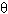= 45°
L = 0.80"
Y = 1.00"
Ind. 2: Subsurface Indications

Rejected*Ind. 1A: BSC,= 45°
d = -2 dB
L = 0.55", Z = 1.72"
X = -0.40", Y = 1.09"
Ind. 1B: TSC,= 45°
d = +3 dB
L = 0.25", Z = 1.82"
X = -0.08", Y = 1.79"
Ind. 2A: TSC,= 45°
d = +9 dB
L = 1.86", Z = 0.08"
X = -2.06", Y = 4.68"
Ind. 2B: BSC,= 45°
d = +10 dB
L = 0.15", Z = 0.05"
X = -0.04", Y = 6.45"
Ind. 3: BSC,= 45°
d = +8 dB
L = 1.10", Z = 2.82"
X = -0.49", Y = 6.68"
Ind. 4: BSC,= 45°
d = +9 dB
L = 4.44", Z = 2.883"
X = +0.56", Y = 11"
Ind. 5: BSC,= 45°
d = +7 dB
L = 1.45", Z = 2.85"
X = -1.08", Y = 16.41"
Ind. 6: BSC,= 45°
d = +8 dB
L = 0.31", Z = 0.07"
X = -0.56", Y = 20.01"
Ind. 7: BSC,= 45°
d = +9 dB
L = 1.19", Z = 2.88"
X = +0.69", Y = 22.86"
Ind. 8: BSC,= 45°
d = +10 dB
L = 2.42", Z = 2.9"
X = +0.84", Y = 27"

FG38K-TF1-TopF-FCM

Accepted

Accepted

Accepted

*Under the provisions of table 6.3 in the AASHTO/AWS D1.5: 2002 Bridge Welding Code (i.e., class B and class C flaws shall be separated by at least 2L), Ind. 1A, Ind. 1B, ... are considered as a single defect, Ind. 2A, Ind. 2B, ... are considered as a single defect, etc.

1 inch = 25.4 mm

### FIELD-TESTING RESULTS

The field testing results are summarized in tables 8 through 12. The first column of tables 8 through 12 indicates the specimen identification code. The second, third, and fourth columns indicate the inspection method performed on each specimen. "Rejected" or "Accepted" indicates that the specimen was either rejected or accepted, respectively, by the employed inspection method. "Not Required" indicates that the owners did not prescribe the particular inspection method for the specimen. In the cases where the specimens were rejected by RT and the owners did not require manual UT inspection, the fabricators used manual UT to determine the defect depth so that efficient repairs could be performed. In tables 8 through 12, "Ind." stands for indication, and the angle (q) indicates the transducer's refracted angle. The indication characteristics in the fourth column (i.e., indication rating (d), length (L), depth (Z), x-position, and y‑position) are obtained from P-scan images. These characteristics are compared with the UT acceptance-rejection criteria in tables 6.3 and 6.4 from the AASHTO/AWS D1.5M/D1.5: 2002 Bridge Welding Code(1) to determine whether the indications are accepted or rejected.

Tables 8 through 12 show that RT was performed on the remaining 44 field specimens according to the owner's requirements. Note that section 6.7.1.2 of the AASHTO/AWS D1.5M/D1.5: 2002 Bridge Welding Code(1) indicates that 100 percent of each joint subjected to calculated tension or stress reversal and 25 percent of each joint subjected to compression or shear should be inspected by manual UT or RT. The P-scan and radiographic images of the rejected field specimens are provided in figures 83 through 96.

The results from the first field test set at HSS are summarized in table 8. Only two specimens were tested in the first set using RT and AUT. Manual UT was not required. RT and AUT accepted both specimens with no rejectable defects.

Table 8. Inspection results of the first field testing at HSS.

Specimen ID

RT

Manual UT

AUT

G20A-TF2-BottF

Accepted

Not Required

Accepted

G21A-TF2-BottF

Accepted

Not Required

Accepted

Table 9. Inspection results of the second field testing at HSS.

Specimen ID

RT

Manual UT

AUT

G3F-CF1-BottF

Accepted

Not Required

Accepted

G3F-CF2-BottF

Accepted

Not Required

Accepted

G3F-TF1-TopF

Accepted

Not Required

Accepted

G3F-TF2-TopF

Accepted

Not Required

Accepted

G4F-TF1-TopF

Accepted

Not Required

Accepted

G4F-TF2-TopF

Accepted

Not Required

Accepted

G3J-TF1-TopF

Accepted

Not Required

Accepted

G3J-CF1-BottF

Accepted

Not Required

Accepted

G2A-CF1-TopF

Accepted

Not Required

Accepted

G2A-CF2-TopF

Accepted

Not Required

Accepted

G2A-CF3-TopF

Accepted

Not Required

Accepted

G2A-CF4-TopF

Accepted

Not Required

Accepted

G5G-TF1-TopF

Rejected
Ind. 1: A-B,
0.13" Slag 0.94" From Edge
(i.e., L = 0.13", Y = 0.94")
Ind. 2: B-C,
0.25" Slag 0.82" From Edge
(i.e., L = 0.25", Y = 19.19")

Not Required
(Accepted Within Code
Ind.1:
L = 0.5", Z = 0.56"
X = 0, Y = 0
Ind. 2:
L = 0.5",
X = 0, Y = 19.0")

Accepted Within Code
Ind. 1: TSC,= 70°
d = +14 dB
L = 0.52", Z = 0.81"
X = -0.05", Y = 0"
Ind. 2: BSC,= 70°
d = +14 dB
L = 1.12", Z = 0.11"
X = -0.22", Y = 18.4"

G2G-CF1-BottF-FCM

Accepted

Rejected

Rejected*Ind. 1A: TSC,= 45°
d = +5 dB
L = 0.58", Z = 1.12"
X = -0.06", Y = 26.21"
Ind. 1B: TSC,= 45°
d = 0 dB
L = 1.50", Z = 1.12"
X = +0.01", Y = 26.93"

*Under the provisions of table 6.3 in the AASHTO/AWS D1.5: 2002 Bridge Welding Code (i.e., class B and class C flaws shall be separated by at least 2L), Ind. 1A, Ind. 1B, ... are considered as a single defect, Ind. 2A, Ind. 2B, ... are considered as a single defect, etc.

1 inch = 25.4 mm

Table 10. Inspection results of the third field testing at HSS.

Specimen ID

RT

Manual UT

AUT

FG4A-TF1-BottF-FCM

Accepted

Accepted

Accepted

FG1A-TF1-BottF-FCM

Accepted

Accepted

Accepted

FG2A-TF1-BottF-FCM

Accepted

Accepted

Accepted

FG3A-TF1-BottF-FCM

Accepted

Accepted

Accepted

FG37K-TF2-TopF-FCM

Accepted

Accepted

Accepted

FG38K-TF3-BottF-FCM

Accepted

Accepted

Accepted

FG26G-TF2-BottF-FCM

Rejected
Ind. 1: A-B,
0.12" Slag 0.59" From B Edge
(i.e., L = 0.12", Y = 11.29")

Accepted Within Code

Accepted Within Code
Ind. 1: BSC,= 70°
d = +7 dB
L = 0.39", Z = 0.76"
X = 0.45", Y = 9.97"

FG16D-TF1-BottF-FCM

Rejected
Ind. 1: A-B, Within Code
0.04" Slag at A
(i.e., L = 0.04", Y = 0")
Ind. 2: A-B,
0.12" Slag 0.98" From B Edge
(i.e., L = 0.12", Y = 11.02")

Accepted Within Code

Accepted Within Code
Ind. 1: BSC,= 70°
d = +14 dB
L = 1.74", Z = 0.69"
X = -0.24", Y = 0.61"
Ind. 2: BSC,= 70°
d = +9 dB
L = 0.72", Z = 0.32"
X = -0.14", Y = 9.56"

FG36K-TF2-TopF-FCM

Rejected
Ind. 1: C-D,
Edge of Plate
(i.e., Y = 33.75")

Rejected
Ind. 1:= 70°
L = 2", Z = 0.5"
X = -0.75", Y = 33.5"
Ind. 2: Subsurface Indications

Rejected*Ind. 1: BSC,= 45°
d = +7 dB
L = 0.83", Z = 0.04"
X = +1.2", Y = 22.55"
Ind. 2A: TSC,= 45°d = +9 dB
L = 1.31", Z = 0"
X = -0.85", Y = 25.55"
Ind. 2B: TSC,= 45°
d = +3 dB
L = 0.70", Z = 0.04"
X = -0.53", Y = 27.60"
Ind. 3A: BSC,= 45°
d = -2 dB
L = 1.31", Z = 0.02"
X = -0.67", Y = 31.18"
Ind. 3B: TSC,= 45°
d = -3 dB
L = 1.43", Z = 0.02"
X = -0.58", Y = 31.78"

FG37K-TF3-BottF-FCM

Accepted

Rejected
Ind. 1:= 45°
L = 1.0", Z = 2.0"
X = +0.50", Y = 26"

Rejected
Ind. 1: BSC,= 45°
d = +6 dB
L = 0.41", Z = 1.85"
X = +0.54", Y = 26.67"

TP2

Rejected
Slags
Ind. 1: L = 0.50", Y = 0.25"
Ind. 2: L = 0.75", Y = 3.50"
Ind. 3: L = 1.13", Y = 7.0"
Ind. 4: L = 1", Y = 10.5"

Rejected
Ind. 1:
d = +5 dB
L = 0.38", Z = 0.92"
X = -0.50", Y = 0"
Ind. 2:
d = +2 dB
L = 0.50", Z = 0.18"
X = +0.31", Y = 3.63"
Ind. 3:
d = -1 dB
L = 0.75", Z = 0.95"
X = -0.13", Y = 7.13"
Ind. 4:
d = +1 dB
L = 0.50", Z = 0.09"
X = -0.38", Y = 10.5"

Rejected
Ind. 1:
d = +4 dB
L = 0.46", Z = 0.97"
X = -0.53", Y = 0"
Ind. 2:
d = +2 dB
L = 0.88", Z = 0.01"
X = +22", Y = 3.17"
Ind. 3:
d = -3 dB
L = 1.12", Z = 0.93"
X = -0.19", Y = 7.0"
Ind. 4:
d = +1 dB
L = 0.89", Z = 0"
X = -0.30", Y = 10.19"

AWS-FCM-02-6A

Rejected

Rejected

Rejected

*Under the provisions of table 6.3 in the AASHTO/AWS D1.5: 2002 Bridge Welding Code (i.e., class B and class C flaws shall be separated by at least 2L), Ind. 1A, Ind. 1B, ... are considered as a single defect, Ind. 2A, Ind. 2B, ... are considered as a single defect, etc.

1 inch = 25.4 mm

Table 11. Inspection results of the fourth field testing at HSS.

Specimen ID RT Manual UT AUT

FBG80-TF1-BottF-FCM

Accepted

Accepted

Accepted

FG1E-TF2-TopF- FCM

Accepted

Accepted

Accepted

FG2E-TF2-TopF-FCM

Accepted

Accepted

Accepted

G11THW-CF1-BottF

Accepted

Not Required

Accepted

G11THW-CF2-BottF

Accepted

Not Required

Accepted

G7UHW-CF1-TopF

Accepted Within Code
Ind. 1: B-C,
Indication 1.31" Right of A
(i.e., Y = 1.31")
Ind. 2: B-C,
Indication 0.5" Left of B (i.e., Y = 17.5")

Not Required

Accepted

FG40M-TF1- Curved-FCM

Accepted

Rejected
Ind. 1:= 45°
d = +1 dB
L = 1.75", Z = 2"
X = +0.38", Y = 12"
Ind. 2:= 45°
d = +5 dB
L = 0.75", Z = 1.38"
X = +0.63", Y = 16"

Rejected*Ind. 1A: BSC,= 45°
d = +8 dB
L = 1.29", Z = 2"
X = +0.49", Y = 10.68"
Ind. 1B: TSC,= 45°
d = +1 dB
L = 1.02", Z = 1.37"
X = +1.10", Y = 11.17"

FG1A-TF2-BottF- FCM

Rejected
Ind. 1: A-B, Slag

Rejected

Rejected*Ind. 1A: TSC,= 60°
d = -1 dB
L = 0.97", Z = 0.49"
X = -1.40", Y = 3.0"
Ind. 1B: TSC,= 70°
d = +6 dB
L = 1.11", Z = 0.72"
X = -1.50", Y = 3.08"

G3VHW-CF1-BottF

Rejected
Ind. 1: A-B,
Indication 3.25" Left of B (i.e., Y = 9.88")

Not Required
(Accepted Within Code)

Accepted Within Code
Ind. 1: TSC,= 70°
d = +4 dB
L = 0.66", Z = 1.52"
X = +1.46", Y = 8.76"

G5VHW-CF1-BottF

Rejected
Ind. 1: B-C,
0.25" Slag 1.06 From C Edge
(i.e., L = 0.25", Y = 25.19")

Not Required
(Accepted Within Code)

Accepted Within Code
Ind. 1: TSC,= 45°
d = +9 dB
L = 0.40", Z = 1.70"
X = +0.71", Y = 24.76"

*Under the provisions of table 6.3 in the AASHTO/AWS D1.5: 2002 Bridge Welding Code (i.e., class B and class C flaws shall be separated by at least 2L), Ind. 1A, Ind. 1B, ... are considered as a single defect, Ind. 2A, Ind. 2B, ... are considered as a single defect, etc.

1 inch = 25.4 mm

Table 12. Inspection results of field testing at Stupp.

Specimen ID

RT

Manual UT

AUT

FS32B1&2-BottF Joint B015

Accepted

Accepted

Accepted

FS45A1&2 Joint T019

Accepted

Accepted

Accepted

FS46A1&2 Joint T021

Accepted

Accepted

Accepted

FS31B1&2 Joint B011

Accepted Within Code

Accepted

Accepted